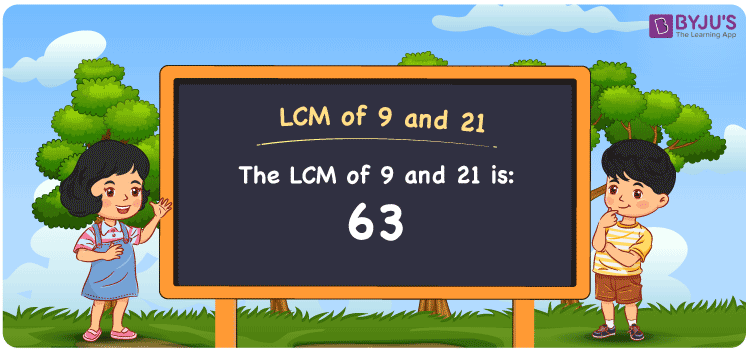Checkout JEE MAINS 2022 Question Paper Analysis : Checkout JEE MAINS 2022 Question Paper Analysis :

# LCM of 9 and 21

LCM of 9 and 21 is 63. Students can learn the concept of the least common multiple of 9 and 21 using common multiples from the study materials at BYJU’S. (9, 18, 27, 36, 45, 54, ….) and (21, 42, 63, 84, 105,….) are the multiples of 9 and 21. The LCM of two numbers can be found using the prime factorisation method, by listing the multiples and division methods.

## What is LCM of 9 and 21?

The answer to this question is 63. The LCM of 9 and 21 using various methods is shown in this article for your reference. The LCM of two non-zero integers, 9 and 21, is the smallest positive integer 63 which is divisible by both 9 and 21 with no remainder.## How to Find LCM of 9 and 21?

LCM of 9 and 21 can be found using three methods:

• Prime Factorisation
• Division method
• Listing the multiples

### LCM of 9 and 21 Using Prime Factorisation Method

The prime factorisation of 9 and 21, respectively, is given by:

9 = 3 x 3 = 3²

21 = 3 x 7 = 3¹ x 7¹

LCM (9, 21) = 63

### LCM of 9 and 21 Using Division Method

We’ll divide the numbers (9, 21) by their prime factors to get the LCM of 9 and 21 using the division method (preferably common). The LCM of 9 and 21 is calculated by multiplying these divisors.

 3 9 21 3 3 7 7 1 7 x 1 1

No further division can be done.

Hence, LCM (9, 21) = 63

### LCM of 9 and 21 Using Listing the Multiples

To calculate the LCM of 9 and 21 by listing out the common multiples, list the multiples as shown below

 Multiples of 9 Multiples of 21 9 21 18 42 27 63 36 84 45 105 54 126 63 147 72 168

The smallest common multiple of 9 and 21 is 63.

Therefore LCM (9, 21) = 63

## Video Lesson on Applications of LCM## LCM of 9 and 21 Solved Examples

What is the LCM if the product of two numbers is 189 and the GCD is 3?

Solution:

It is given that

Product of two numbers = 189

GCD = 3

We know that

LCM x GCD = Product of two numbers

LCM = Product/GCD

LCM = 189/3

LCM = 63

Hence, the LCM is 63.

## Frequently Asked Questions on LCM of 9 and 21

### What is the LCM of 9 and 21?

The LCM of 9 and 21 is 63. To get the LCM value, we should first find the multiples of 9 and 21 and the value which is divisible by 9 and 21 has to be determined.

### What methods can be used to find the LCM value of 9 and 21?

The methods which can be used to find the LCM of 9 and 21 are Division Method, Prime Factorization Method and Listing multiples.

### Determine the GCF if the LCM of 9 and 21 is 63.

LCM x GCF = 9 x 21

Given

LCM of 9 and 21 = 63

63 x GCF = 189

GCF = 189/63 = 3